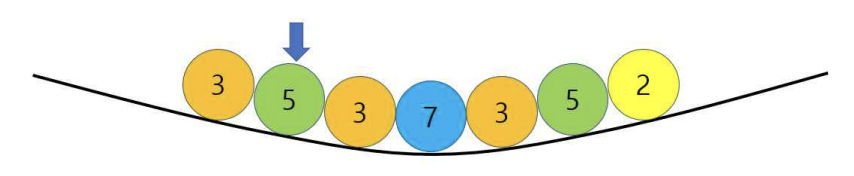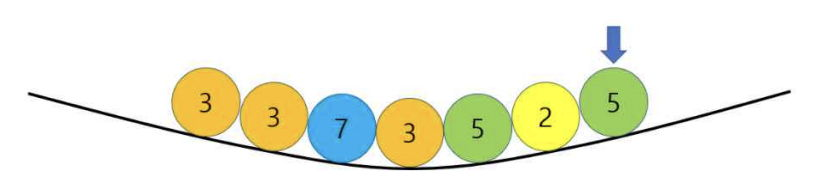시간 제한메모리 제한제출정답맞힌 사람정답 비율
1 초 512 MB342745827.103%

## 문제

Balls are placed on a curved structure as shown in Figure A.1, and each ball has a number written on it. Due to the law of gravity, all balls naturally gather near the lowest spot of the structure. The permitted action to change the position of the balls is to “pick a ball, lift it up, and place it on the far left or on the far right”. This action will naturally change the location of some balls due to the law of gravity.Figure A.1

Figure A.2 shows how the locations of the balls change when the marked ball 5 was picked up in Figure A.1 and placed on the far right.Figure A.2

When n balls are arranged on a curved structure we want to sort the numbers written on the balls in non- decreasing order by repeating the permitted actions. In Figure A.2, if ball 7 is picked up and placed on the far right and ball 2 far left, all the numbers on balls are sorted in non-decreasing order.

Given an arrangement of n balls, write a program which helps to rearrange all the numbers written on the balls in non-decreasing order by selecting minimum number of balls and relocating them through the permitted actions.

## 입력

Your program is to read from standard input. The input starts with a line containing an integer n (1 ≤ n ≤ 105), which denotes the number of balls. Each of the following n lines contains an integer v (1 ≤ v ≤ 109), which is the number written on a ball. Notice that some balls may have the same number written on them.

## 출력

Your program is to write to standard output. Print exactly one line. The line should contain the minimum number of balls picked up and relocated to align all the numbers written on the n balls in non-decreasing order.

## 예제 입력 1

4
21
44
39
41


## 예제 출력 1

1


## 예제 입력 2

7
11
12
25
16
11
18
25


## 예제 출력 2

2


## 출처

• 데이터를 추가한 사람: kyo20111